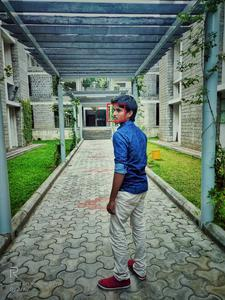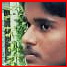Related Articles

# Cropping Faces from Images using OpenCV – Python

• Last Updated : 13 Jan, 2021

Opencv is a python library mainly used for image processing and computer vision. In this article first, we detect faces after that we crop the face from the image. Face detection is the branch of image processing that uses to detect faces.

We will use a pre-trained Haar Cascade model to detect faces from the image.  A haar cascade is the object detection method used to detect objects from the image. This algorithm was trained by numerous images. You can download the face haar cascade file by click here.

Let’s Implementation in stepwise:

Step 1: In this step, we will read the image and converted it into grayscale.

## Python3

 `import` `cv2`` ` `# Read the input image``img ``=` `cv2.imread(``'mpw.jpeg'``)`` ` `# Convert into grayscale``gray ``=` `cv2.cvtColor(img, cv2.COLOR_BGR2GRAY)`

Explanation: In this code first we import our opencv library using import cv2. cv2.imread() method loads the image from the given path(ex:mpw.jpeg or filepath/mpw.jpeg) After loading the image we convert the image to a grayscale image using COLOR_BGR2GRAY.

Step 2: Use the Haar Cascade model to detect faces from the image.

## Python3

 `face_cascade ``=` `cv2.CascadeClassifier(``'haarcascade_frontalface_alt2.xml'``)`` ` `# Detect faces``faces ``=` `face_cascade.detectMultiScale(gray, ``1.1``, ``4``)`

• Gray: input image(gray scale image)
• 1.1: scale factor, it specifies how much the image size is reduced with each scale. It improves detection.
• 4: MinNeighbours, specifies how many neighbors each candidate rectangle should have to retain.

Step 3: Find the face in the image.

## Python3

 `for` `(x, y, w, h) ``in` `faces:``    ``cv2.rectangle(img, (x, y), (x``+``w, y``+``h), ``                  ``(``0``, ``0``, ``255``), ``2``)``     ` `    ``faces ``=` `img[y:y ``+` `h, x:x ``+` `w]``    ``cv2.imshow(``"face"``,faces)``    ``cv2.imwrite(``'face.jpg'``, faces)`` ` `cv2.imshow(``'img'``, img)``cv2.waitKey()`

Explanation: x,y are pixel location of faces, w,h are width and height of faces. cv2.rectangle() function used for draw rectangle over the detected object, img is input image, (x,y),(x+w, y+h) are locations of rectangle,(0,0,255) is color of a rectangle this argument gets passed as a tuple for BGR,we would use (0,0,255) for red, 2 is thickness of rectangle.

Below is the full implementation:

Image Used –input image

## Python3

 `import` `cv2`` ` `# Read the input image``img ``=` `cv2.imread(``'mpw.jpeg'``)`` ` `# Convert into grayscale``gray ``=` `cv2.cvtColor(img, cv2.COLOR_BGR2GRAY)`` ` `# Load the cascade``face_cascade ``=` `cv2.CascadeClassifier(``'haarcascade_frontalface_alt2.xml'``)`` ` `# Detect faces``faces ``=` `face_cascade.detectMultiScale(gray, ``1.1``, ``4``)`` ` `# Draw rectangle around the faces and crop the faces``for` `(x, y, w, h) ``in` `faces:``    ``cv2.rectangle(img, (x, y), (x``+``w, y``+``h), (``0``, ``0``, ``255``), ``2``)``    ``faces ``=` `img[y:y ``+` `h, x:x ``+` `w]``    ``cv2.imshow(``"face"``,faces)``    ``cv2.imwrite(``'face.jpg'``, faces)``     ` `# Display the output``cv2.imwrite(``'detcted.jpg'``, img)``cv2.imshow(``'img'``, img)``cv2.waitKey()`

Output:Detected facecropped image

Attention geek! Strengthen your foundations with the Python Programming Foundation Course and learn the basics.

To begin with, your interview preparations Enhance your Data Structures concepts with the Python DS Course. And to begin with your Machine Learning Journey, join the Machine Learning – Basic Level Course

My Personal Notes arrow_drop_up